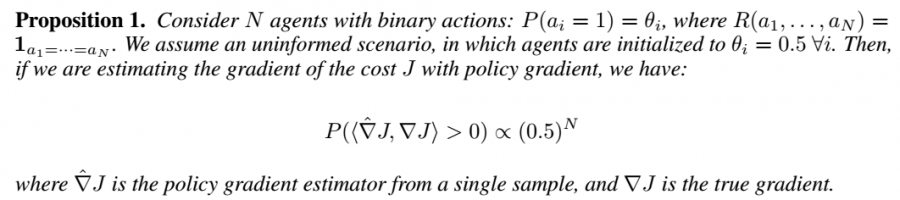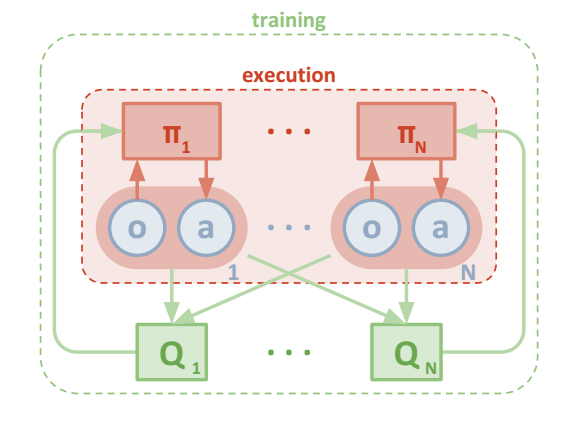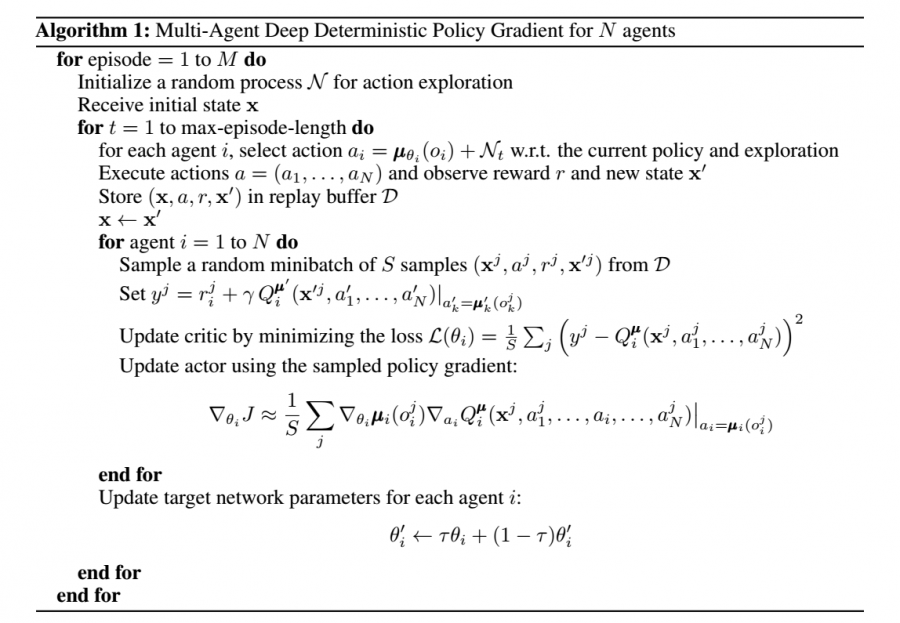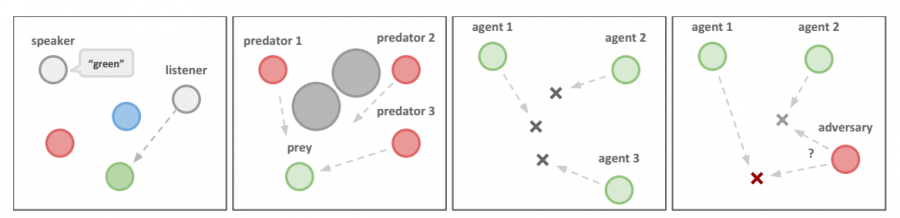# 多智能体强化学习算法

## MADDPG：Multi-Agent Actor-Critic for Mixed Cooperative-Competitive Environments

Posted by MY on August 13, 2020

## 一、问题

• Independent Q-learning: 每个智能体都在随着训练而改变策略，导致整个环境的不稳定性。因此智能体存到replay buffer的经验都不能用。## 二、解法

### 2.1 Multi-Agent Actor CriticActor Critic的策略梯度公式为：$\nabla_{\theta_{i}} J\left(\theta_{i}\right)=E_{s \sim p^{u}, a_{i} \sim \pi_{i}}\left[\nabla_{\theta_{i}} \log \pi_{i}\left(a_{i} \| o_{i}\right) Q_{i}^{\pi}\left(o_{i}, a_{i}\right)\right]$

$\nabla_{\theta_{i}} J\left(\theta_{i}\right)=\mathbb{E}_{s \sim p^{\mu}, a_{i} \sim \pi_{i}}\left[\nabla_{\theta_{i}} \log \pi_{i}\left(a_{i} | o_{i}\right) Q_{i}^{\pi}\left(\mathrm{x}, a_{1}, \ldots, a_{N}\right)\right]$

$\nabla_{\theta_{i}} J\left(\boldsymbol{\mu}_{i}\right) = \mathbb{E}_{\mathbf{x}, a \sim \mathcal{D}}\left[\left.\nabla_{\theta_{i}} \boldsymbol{\mu}_{i}\left(a_{i} | o_{i}\right) \nabla_{a_{i}} Q_{i}^{\boldsymbol{\mu}}\left(\mathbf{x}, a_{1}, \ldots, a_{N}\right)\right|_{a_{i}=\boldsymbol{\mu}_{i}}\left(o_{i}\right)\right]$

$\mathcal{L}\left(\theta_{i}\right)=\mathbb{E}_{\mathbf{x}, a, r, \mathbf{x}^{\prime}}\left[\left(Q_{i}^{\mu}\left(\mathbf{x}, a_{1}, \ldots, a_{N}\right)-y\right)^{2}\right], \quad y=r_{i}+\left.\gamma Q_{i}^{\mu^{\prime}}\left(\mathbf{x}^{\prime}, a_{1}^{\prime}, \ldots, a_{N}^{\prime}\right)\right|_{a_{j}^{\prime}=\boldsymbol{\mu}_{j}^{\prime}\left(o_{j}\right)}$

### 2.2 Inferring Policies of Other Agents

$\mathcal{L}\left(\phi_{i}^{j}\right)=-\mathbb{E}_{o_{j}, a_{j}}\left[\log \hat{\boldsymbol{\mu}}_{i}^{j}\left(a_{j} | o_{j}\right)+\lambda H\left(\hat{\boldsymbol{\mu}}_{i}^{j}\right)\right]$

$\hat{y}=r_{i}+\gamma Q_{i}^{\boldsymbol{\mu}^{\prime}}\left(\mathbf{x}^{\prime}, \hat{\boldsymbol{\mu}}_{i}^{\prime 1}\left(o_{1}\right), \ldots, \boldsymbol{\mu}_{i}^{\prime}\left(o_{i}\right), \ldots, \hat{\boldsymbol{\mu}}_{i}^{\prime N}\left(o_{N}\right)\right)$

### 2.3 Agents with Policy Ensembles

$J_{e}\left(\boldsymbol{\mu}_{i}\right)=\mathbb{E}_{k \sim \operatorname{unif}(1, K), s \sim p^{\mu}, a \sim \boldsymbol{\mu}_{i}^{(k)}}\left[R_{i}(s, a)\right]$

$\nabla_{\theta_{i}^{(k)}} J_{e}\left(\boldsymbol{\mu}_{i}\right)=\frac{1}{K} \mathbb{E}_{\mathbf{x}, a \sim \mathcal{D}_{i}^{(k)}} \left[\left.\nabla_{\theta_{i}^{(k)}} \boldsymbol{\mu}_{i}^{(k)}\left(a_{i} | o_{i}\right) \nabla_{a_{i}} Q^{\boldsymbol{\mu}_{i}}\left(\mathbf{x}, a_{1}, \ldots, a_{N}\right)\right|_{a_{i}=\boldsymbol{\mu}_{i}^{(k)}}\left(o_{i}\right)\right]$## 三、实验内容## 四、缺点

• 每一个Critic需要观测到所有的agent的状态和动作，对于大量不确定agent的场景，不是特别实用
• 当agent数量特别多的时候，状态空间太过于巨大
• 每一个agent都对应了一个actor和一个critic，数量多的时候，存在大量的模型。

## 五、优点

• 对于集中训练分步执行的方法是一种完善
• 是多智能体环境下的开创性工作。

##### Share